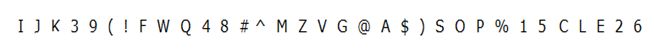# SBI PO Prelims Reasoning (Day-05)

Dear Aspirants, Our IBPS Guide team is providing new series of Reasoning Questions for SBI PO 2020 Prelims so the aspirants can practice it on a daily basis. These questions are framed by our skilled experts after understanding your needs thoroughly. Aspirants can practice these new series questions daily to familiarize with the exact exam pattern and make your preparation effective.

Start Quiz

Order and ranking

Directions (1-3): Study the following information carefully and answer the questions given below:

Seven cities – V, L, P, R, G, D and X have different number of lakes. City P has more number of lakes than City V but less number of lakes than City R.  Only one city has less number of lakes than City D. City L has less number of lakes than City X but more number of lakes than City V. City X has more number of lakes than City R. City V does not have the least number of lakes. City R has more number of lakes than L.

1) Which of the following city has maximum number of lake?

A) P

B) L

C) R

D) X

E) None of these

2) How many cities have more number of lake than City P?

A) One

B) Two

C) Three

D) Four

E) Cannot be determined

3) Which are the two cities have less number of lakes than City V?

A) P, L

B) R, P

C) X, P

D) G, D

E) None of these

Misc

4) How many meaningful English words can be formed with the letter D, P, A, U, E, T using each letter only once in each word?

A) One

B) Two

C) Three

D) Four

E) None of these

Inequality

Directions (5-6): In each question, relationships between different elements are shown in the statements. The statements are followed by some conclusions. Study the conclusions based on the given statements and select the appropriate answer.

5) Statements:

L ≥ M = N > G ≤ H = J;         B ≥ C = N < O ≤ P < I;

Conclusions:

I) C < L

II) J > I

III) I > G

IV) M=J

A) Only I follow

B) Only III follow

C) Either I or IV follows

D) Only III and Neither II nor IV follows

E) None of these

6) Statements:

R > N = W ≤ D < K;  V < H ≥ O = T;   S ≤ N = H > A

Conclusions:

I) R > T

II) S < K

III) V < D

IV) T ≤ D

A) Only III follow

B) Only II and III follow

C) All follows

D) Only I and III follow

E) None follows

Alphanumeric series

Directions (7-10): Study the given information and answer the questions:7) How many symbols are there which is immediately followed by vowels and preceded by consonants?

A) None

B) 1

C) 2

D) 3

E) None of these

8) If all the numbers from the above sequence are dropped, which of the following element is 8th to the right of the 15th from the left end?

A) 1

B) 5

C) C

D) L

E) None of these

9) How many consonants are there immediately followed by number and preceded by consonant?

A) None

B) 1

C) 2

D) 3

E) None of these

10) If all the symbols from the above sequence are dropped, which of the following element is 19th from the right end?

A) Q

B) W

C) F

D) 4

E) None of these

Directions (1-3) :

X > R>P/L>P/L>V>D>G

X > R>P/L>P/L>V>D>G

X > R>P/L>P/L>V>D>G

UPDATE

Directions (5-6) :

Directions (7-10) :

I J K 3 9 ( ! F W Q 4 8 # ^ M Z V G @ A \$ ) S O P % 1 5 C L E 2 6

I J K  ( ! F W Q  # ^ M Z V G @ A \$ ) S O P %  C L E

J K 3 9 ( ! F W Q 4 8 # ^ M Z V G @ A\$ ) S O P % 1 5 C L E 2 6